## How to calculate a future date based on a given date in Excel?

If you need to add a number of days to a given date to calculate the future date, how could you deal with it in Excel?

Calculate a future date based on a given date with formula

Calculate a future date exclude weekends based on a given date with formula

Calculate a future date exclude weekends and holidays based on a given date with formula

Calculate a future date based on a given date with a powerful feature

#### Calculate a future date based on a given date with formula

To get a future date which is 100 days later based on current date, please apply the following simple formula:

Enter the following formula into a blank cell to locate the result, and then drag the fill handle down to the cells for getting the future date as following screenshot shown:

=A2+100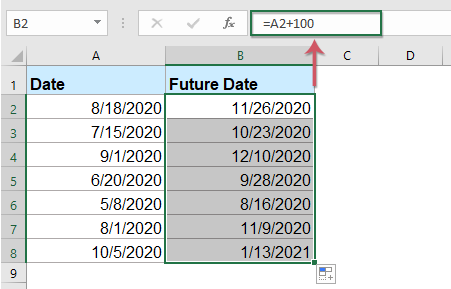#### Calculate a future date exclude weekends based on a given date with formula

If you want to calculate the future date exclude weekends, please use this formula:

1. Type the below formula into a blank cell, and then drag the fill hanlde to the cells you want to apply the formula, and five-digit number will be displayed, see screenshot:

=WORKDAY(A2,100)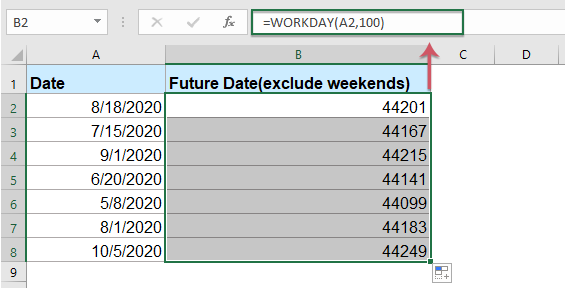2. Then you should convert the five-digit number to date by clicking Short Date from the General drop-down list in the Home tab, see screenshot: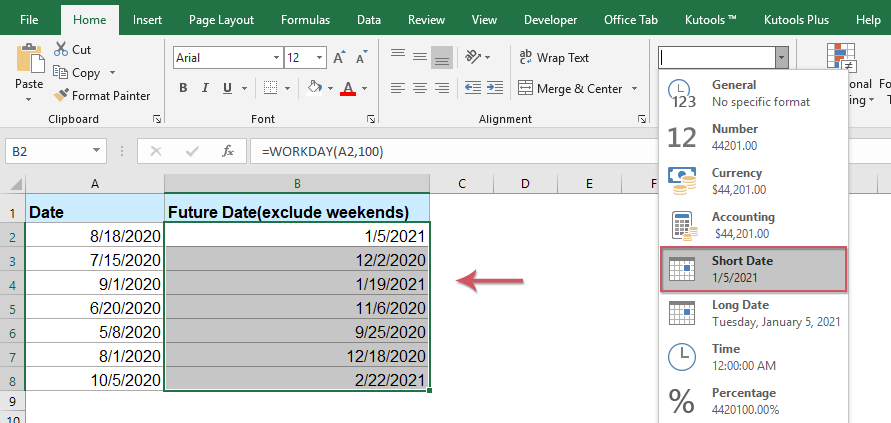#### Calculate a future date exclude weekends and holidays based on a given date with formula

1. Enter the below formula into a blank cell, and drag the fill hanlde to apply the formula to other cells, then some numbers are displayed, see screenshot:

=WORKDAY(A2,100,\$E\$2:\$E\$5)

Note: In the above formula, A2 is the current date that you want to calculate future date based on, 100 is the number of days you want to add to the date, E2:E5 is the holiday cells which needed to be excluded, please change them to your need.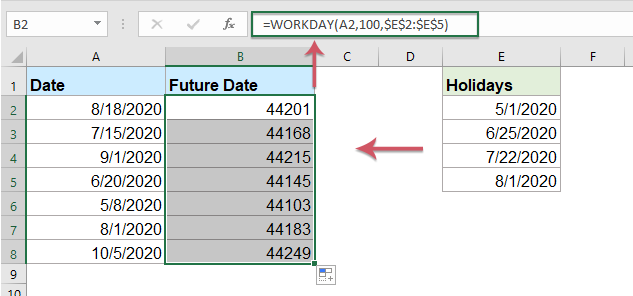2. Then select the formula cell, and click Short Date from the General drop-down list under the Home tab to convert the number to date format, see screenshot: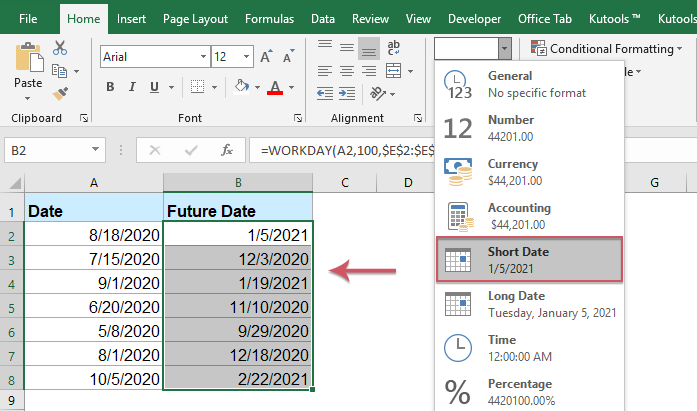#### Calculate a future date based on a given date with a powerful feature

If you need to calculate a future date by adding a number of days, weeks, months or years, the Kutools for Excel’s Date & Time helper function can do you a favor.

Tips:To apply this Date & Time helper feature, firstly, you should download the Kutools for Excel, and then apply the feature quickly and easily.

After installing Kutools for Excel, please do as follows:

1. Select a cell where you want to locate the calculated result, and then click Kutools > Formula Helper > Date & Time helper, see screenshot: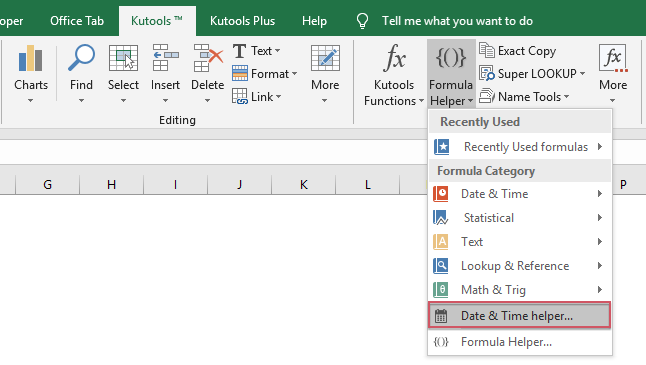2. In the Date & Time Helper dialog box, do the following operations:

• Select Add option from the Type section;
• Then click to select a cell that you want to calculate a future date based on;
• At last, enter the numbers of years, months, weeks or days that you want to add for the given date.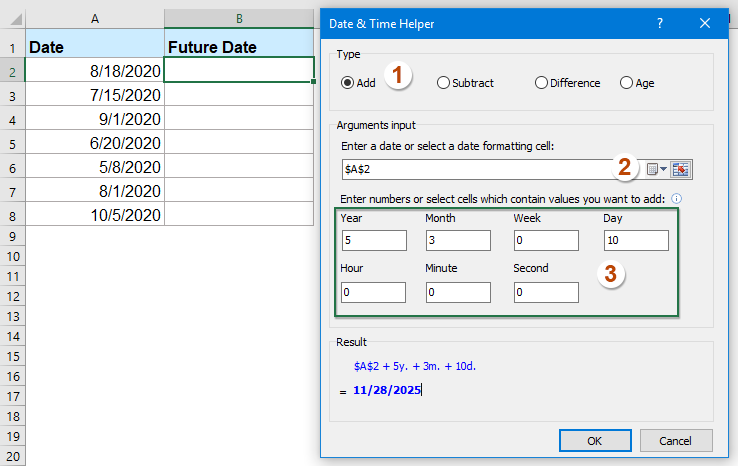3. Then click OK button, and you will get the future date. And then, drag the fill hanlde to fill the formula to other cells you need, see screenshot: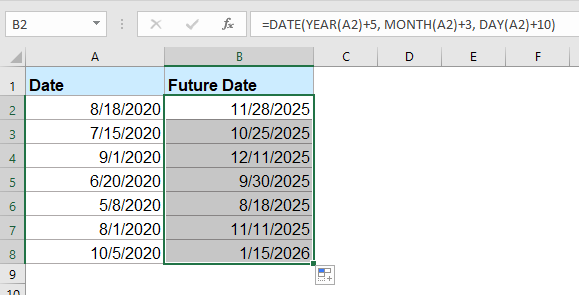#### More relative date and time articles:

• Calculate Hours Between Times After Midnight In Excel
• Supposing you have a time table to record your work time, the time in Column A is the start time of today and time in Column B is the end time of the following day. Normally, if you calculate the time difference between the two times by directly minus "=B2-A2", it will not display the correct result as left screenshot shown. How could you calculate the hours between two times after midnight in Excel correctly?
• Calculate Working Hours Per Month In Excel
• Normally, most of us may work 8 hours a day, 5 days a week. Here, I want to get the total working hours per month for calculating the salary. How to calculate the total working hours per month in Excel?
• Calculate Hours Worked And Minus Lunch Time In Excel
• As a company employee, we need punch in and punch out every work day, counting the total worked time and minus the lunch time of a day can help to calculate salary according to the time. For example, I have the following daily attendance record which contains log in, log out and lunch start, lunch end times in a worksheet, now, I want to calculate the total hours worked but minus lunch time each day. To finish this job, I can introduce some formulas for you.

### Best Office Productivity Tools

 Popular Features: Find, Highlight or Identify Duplicates   |  Delete Blank Rows   |  Combine Columns or Cells without Losing Data   |   Round without Formula ... Super Lookup: Multiple Criteria VLookup  |   Multiple Value VLookup  |   VLookup Across Multiple Sheets   |   Fuzzy Lookup .... Advanced Drop-down List: Quickly Create Drop Down List   |  Dependent Drop Down List   |  Multi-select Drop Down List .... Column Manager: Add a Specific Number of Columns  |  Move Columns  |  Toggle Visibility Status of Hidden Columns  |  Compare Ranges & Columns ... Featured Features: Grid Focus   |  Design View   |   Big Formula Bar   |  Workbook & Sheet Manager   |  Resource Library (Auto Text)   |  Date Picker   |  Combine Worksheets   |  Encrypt/Decrypt Cells   |  Send Emails by List   |  Super Filter   |   Special Filter (filter bold/italic/strikethrough...) ... Top 15 Toolsets:  12 Text Tools (Add Text, Remove Characters, ...)   |   50+ Chart Types (Gantt Chart, ...)   |   40+ Practical Formulas (Calculate age based on birthday, ...)   |   19 Insertion Tools (Insert QR Code, Insert Picture from Path, ...)   |   12 Conversion Tools (Numbers to Words, Currency Conversion, ...)   |   7 Merge & Split Tools (Advanced Combine Rows, Split Cells, ...)   |   ... and more

Supercharge Your Excel Skills with Kutools for Excel, and Experience Efficiency Like Never Before. Kutools for Excel Offers Over 300 Advanced Features to Boost Productivity and Save Time.  Click Here to Get The Feature You Need The Most...#### Office Tab Brings Tabbed interface to Office, and Make Your Work Much Easier

• Enable tabbed editing and reading in Word, Excel, PowerPoint, Publisher, Access, Visio and Project.
• Open and create multiple documents in new tabs of the same window, rather than in new windows.
• Increases your productivity by 50%, and reduces hundreds of mouse clicks for you every day!No ratings yet. Be the first to rate!
This comment was minimized by the moderator on the site
hi, how can i used excel to calculate future dates from a given date.
I want to calculate range ie, 11-13 weeks from 1/1/2024
can someone help with formula
thanks
This comment was minimized by the moderator on the site
very to easy use
This comment was minimized by the moderator on the site
Hi there, thank you for sharing your knowledge. I need to take a current contract expiry date and monitor it with a future date in mind. The task is to consider expiry of contract within a 36 month period, 24 month period and a 12 month period. These would be milestone dates that trigger my work.
There are no comments posted here yet